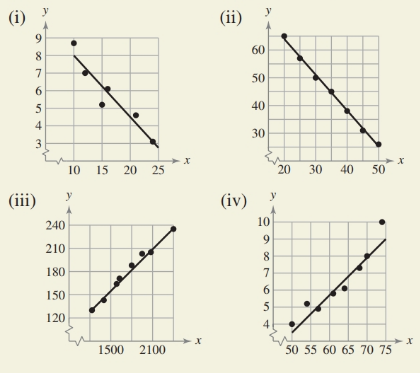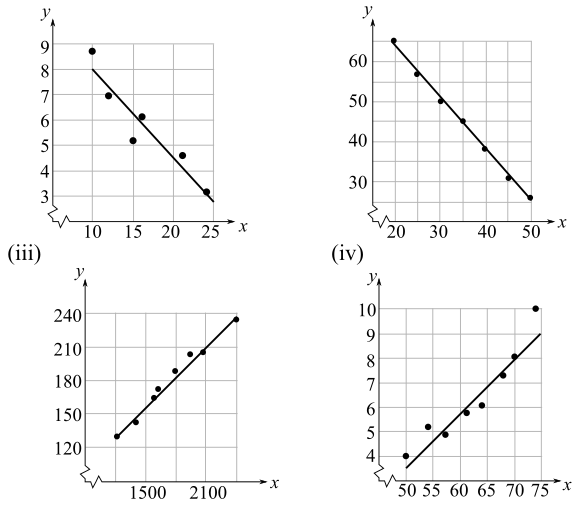Chapter 7.7, Problem 18E### Calculus: An Applied Approach (Min...

10th Edition
Ron Larson
ISBN: 9781305860919

#### Solutions

Chapter
Section### Calculus: An Applied Approach (Min...

10th Edition
Ron Larson
ISBN: 9781305860919
Textbook Problem
1 views

# HOW DO YOU SEE IT? Match the regression equation with the appropriate graph. Explain your reasoning. (Note that the x- and y-axes are broken.)(a) y = 0.22 x − 7.5 (b) y = − 0.35 x + 11.5 (c) y = 0.09 x + 19.8 (d) y = − 1.29 x + 89.8(a)

To determine

The correct regression line equation with the appropriate graph given as below,Explanation

Given Information:

The regression lines are given below,

(a) y=0.22x75(b) y=0.35x+11.5(c) y=0.09x+19.8(d)y=1.29x+89.8

And, the provided graph is given below,

Consider the given information,

Now, to draw the graph of option a regression line.

Follow the Given below steps on TI-83 calculator.

Step 1: Press ON key.

Step 2: Press Y= key to enter the function as Y=0.22X75

(b)

To determine

The correct regression line equation with the appropriate graph given as below,(c)

To determine

The correct regression line equation with the appropriate graph given as below,(d)

To determine

The correct regression line equation with the appropriate graph given as below,### Still sussing out bartleby?

Check out a sample textbook solution.

See a sample solution

#### The Solution to Your Study Problems

Bartleby provides explanations to thousands of textbook problems written by our experts, many with advanced degrees!

Get Started